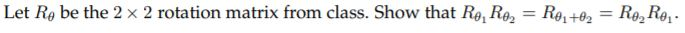Home / Answered Questions / Other / let-r-be-the-2-2-rotation-matrix-from-class-show-that-r-1r-2-r-1-2-r-2r-1-aw294

# (Solved): Let RÎ¸ Be The 2 Ã— 2 Rotation Matrix From Class. Show That RÎ¸1RÎ¸2 = RÎ¸1+Î¸2 = RÎ¸2RÎ¸1 ....

Let RÎ¸ be the 2 Ã— 2 rotation matrix from class. Show that RÎ¸1RÎ¸2 = RÎ¸1+Î¸2 = RÎ¸2RÎ¸1 .Let Ro be the 2 x 2 rotation matrix from class. Show that Ro, Re, = R0 +03 = Re, Re.

We have an Answer from Expert